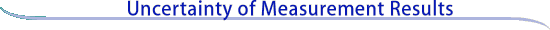Essentials of expressing measurement uncertainty

This is a brief summary of the method of evaluating and expressing uncertainty in measurement adopted widely by U.S. industry, companies in other countries, NIST, its sister national metrology institutes throughout the world, and many organizations worldwide. These "essentials" are adapted from NIST Technical Note 1297 (TN 1297), prepared by B.N. Taylor and C.E. Kuyatt and entitled Guidelines for Evaluating and Expressing the Uncertainty of NIST Measurement Results, which in turn is based on the comprehensive International Organization for Standardization (ISO) Guide to the Expression of Uncertainty in Measurement. Users requiring more detailed information may access TN 1297 online, or if a comprehensive discussion is desired, they may purchase the ISO Guide.

Background information on the development of the ISO Guide, its worldwide adoption, NIST TN 1297, and the NIST policy on expressing measurement uncertainty is given in the section International and U.S. perspectives on measurement uncertainty.

To assist you in reading these guidelines, you may wish to consult a short glossary. Additionally, a companion publication to the ISO Guide, entitled the International Vocabulary of Basic and General Terms in Metrology, or VIM, gives definitions of many other important terms relevant to the field of measurement. Users may also purchase the VIM.

Basic definitions

Measurement equation

The case of interest is where the quantity Y being measured, called the measurand, is not measured directly, but is determined from N other quantities X1, X2, . . . , XN through a functional relation f, often called the measurement equation:

 Y = f(X1, X2, . . . , XN) (1)

Included among the quantities Xi are corrections (or correction factors), as well as quantities that take into account other sources of variability, such as different observers, instruments, samples, laboratories, and times at which observations are made (e.g., different days). Thus, the function f of equation (1) should express not simply a physical law but a measurement process, and in particular, it should contain all quantities that can contribute a significant uncertainty to the measurement result.

An estimate of the measurand or output quantity Y, denoted by y, is obtained from equation (1) using input estimates x1, x2, . . . , xN for the values of the N input quantities X1, X2, . . . , XN. Thus, the output estimate y, which is the result of the measurement, is given by

 y = f(x1, x2, . . . , xN). (2)

For example, as pointed out in the ISO Guide, if a potential difference V is applied to the terminals of a temperature-dependent resistor that has a resistance R0 at the defined temperature t0 and a linear temperature coefficient of resistance b, the power P (the measurand) dissipated by the resistor at the temperature t depends on V, R0, b, and t according to

 P = f(V, R0, b, t) = V2/R0[1 + b(t - t0)]. (3)Classification of uncertainty components The uncertainty of the measurement result y arises from the uncertainties u (xi) (or ui for brevity) of the input estimates xi that enter equation (2). Thus, in the example of equation (3), the uncertainty of the estimated value of the power P arises from the uncertainties of the estimated values of the potential difference V, resistance R0, temperature coefficient of resistance b, and temperature t. In general, components of uncertainty may be categorized according to the method used to evaluate them. Type A evaluation method of evaluation of uncertainty by the statistical analysis of series of observations, Type B evaluation method of evaluation of uncertainty by means other than the statistical analysis of series of observations. Representation of uncertainty components Standard Uncertainty Each component of uncertainty, however evaluated, is represented by an estimated standard deviation, termed standard uncertainty with suggested symbol ui, and equal to the positive square root of the estimated variance Standard uncertainty: Type A An uncertainty component obtained by a Type A evaluation is represented by a statistically estimated standard deviation si, equal to the positive square root of the statistically estimated variance si2, and the associated number of degrees of freedom vi. For such a component the standard uncertainty is ui = si. Standard uncertainty: Type B In a similar manner, an uncertainty component obtained by a Type B evaluation is represented by a quantity uj , which may be considered an approximation to the corresponding standard deviation; it is equal to the positive square root of uj2, which may be considered an approximation to the corresponding variance and which is obtained from an assumed probability distribution based on all the available information. Since the quantity uj2 is treated like a variance and uj like a standard deviation, for such a component the standard uncertainty is simply uj. Continue to Evaluating uncertainty components: Type A